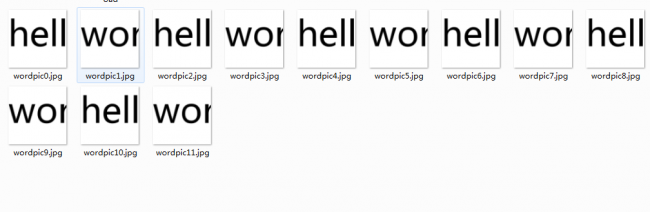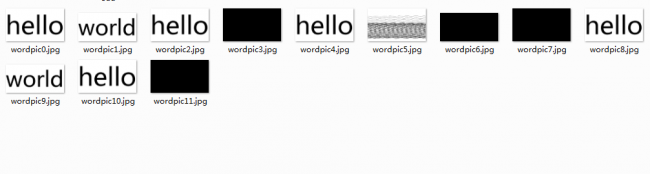#### Howdy, Stranger!

We are about to switch to a new forum software. Until then we have removed the registration on this forum.

# Unexpected Results of the function createGraphics

edited March 2016

I am working on a small demo about creating text clouds, and got stuck in the createGraphics().

Here is my Code:

``````    PImage pic;
PFont font;
PGraphics pg;
PGraphics manyC,finalSave;
String[] words={"hello","world","hello","world","hello","world","hello","world","hello","world","hello","world"};
PGraphics[] wordPic;

void setup(){
size(1800,1800);
pic.filter(THRESHOLD,0.9);
pic.resize(1800,0);
pic.save("D://sample.png");

font=createFont("微软雅黑",240);
textFont(font);
float strHt=textAscent()+textDescent();
manyC      = createGraphics(pic.width,pic.height);
finalSave  = createGraphics(pic.width,pic.height);
//finalSave.smooth();

wordPic=new PGraphics[words.length];
int[] w =  new int;
w = 400;
w = 400;
w = 400;
w = 400;
w = 400;
w = 400;
w = 400;
w = 400;
w = 400;
w = 400;
w = 400;
w = 400;
w = 400;
w = 400;

for(int i=0;i<wordPic.length;i++){

int wid = (int)textWidth(words[i]);
println(wid);
wordPic[i] = createGraphics(w[i],400);
wordPic[i].beginDraw();
wordPic[i].background(255);
wordPic[i].textFont(font);
wordPic[i].textAlign(LEFT,TOP);
wordPic[i].fill(color(0,0,0));
wordPic[i].text(words[i],0,0);
wordPic[i].endDraw();

if(wordPic[i].width>=width){
wordPic[i].resize(width,0);
}
}

for(int i=0; i<wordPic.length;i++){
wordPic[i].save("D://wordpic"+i+".jpg");
}
println("over");
}
``````

the output is like that:so far so good. However, if I adjust the value of w[], which changes the width value when calling the function createGraphics(), the unexpected result comes out!All I did was changing some values of w[], like 800,1200.

well, I'm confused.

I'm trying to adjust the graphics width, like this: wordPic[i] = createGraphics((int)textWidth(words[i]),(int)strHt); so I can handle them as small objects of the text Cloud.

And clearly, the results are not good enough.

So, is there anyone could help me about this problem? tell me why this will happen, or how can I leave this problem alone and go ahead for my work?

Tagged: